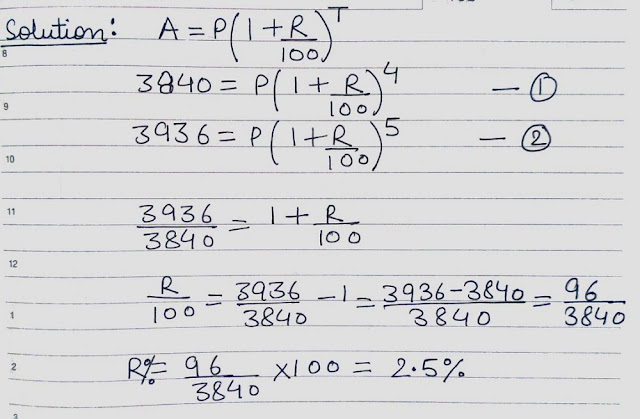Compound Interest Notes

Ashish

Staff member
Compound Interest
Let Principal = P, Rate = R% per annum, Time = n years.
Amount=Principal+Compound Interest

1. When interest is compound Annually2. When interest is compounded Half Yearly3. When interest is compounded Quarterly4. When the interest is compounded monthy5. When interest is compounded Annually but time is in fraction6. When Rates are different for different years, say R1%, R2%, R3% for 1st, 2nd and 3rd year respectively.7. Present worth of Rs. x due n years hence is given by:8. When CI is compounded annually, the ratio of SI and CI at the same rate% per annum and for the same time period is given by=9. The simple interest on a certain sum for 2 years is Rs x and the compound interest is Rs y. Then:
a) Rate of interest=b) Sum=10. If the difference between CI & SI on a certain sum at r% per annum for 2 years is Rs x, then Principal=11. If the difference between CI & SI on a certain sum at r% per annum for 3 years is Rs x, then Principal=12. The ratio of CI to SI on a certain sum at r% for 2 years is=13. A certain sum of money invested at compound interest, compounded yearly, becomes Rs A1 in n years and Rs A2 in (n+1) years. Then:
a) Rate of Interest=b) Principal=14. If the population of a town decreases r% per annum then population after n years is=15. If any sum at the rate of compound interest becomes x1 times in n1 years and x2 times in n2 years, then
Rate=16. The difference of CI and SI for n years at the rate of interest r% on a sum Rs P is=Ashish

Staff member
Question Type 1: Based on Compound Interest Formula

Example:
In what time will Rs 1000 becomes Rs 1331 at 10% per annum compounded annualy?
a) 3 years
b) 2.5 years
c) 2 years
d) 3.5 years
Solution:Ashish

Staff member
Question Type 2: Based on Both Compound Interest & Simple Interest

Example:

The compound interest on a certain sum of money at 5% for 2 years is Rs 328. The simple interest on that sum at the same rate and for the same period of time will be:
a) Rs 320
b) Rs 322
c) Rs 325
d) Rs 326
Solution:Ashish

Staff member
Question Type 3: Difference between Compound Interest & Simple Interest

Example:

If the difference between the compound interest and simple interest on a sum at 5% rate of interest per annum for three years is Rs 36.60, then the sum
is:
a) Rs 8000
b) Rs 8400
c) Rs 4400
d) Rs 4800
Solution:

Example:
The difference between the compound interest and simple interest on Rs 2500 for 2 years at 4% per annum is?
a) Rs 40
b) Rs 45
c) Rs 14
d) Rs 4
Solution:

Example:
If the difference between the simple and compound interests on a sum of money for 2 years at 4% per annum is Rs 80, the sum is:
a) Rs 5000
b) Rs 50000
c) Rs 10000
d) Rs 1000
Solution:

Ashish

Staff member
Question Type 4: If the amount becomes "N" times of the sum after "T" years at Compound Interest

Example:

A sum of money becomes eight times of itself in 3 years at compound interest. The rate of interest is:
a) 100%
b) 80%
c) 20%
d) 10%
Solution:Example:
A sum of money at compound interest doubles itself in 15 years. It will become eight times of itself in :
a) 45 years
b) 48 years
c) 54 years
d) 60 years
Solution:Ashish

Staff member
Question Type 5: A Sum of money amounts to Rs X1 in T1 years and to Rs X2 in T2 years at Compound Interest

Example:

An amount of money at compound interest grows upto Rs 3,840 in 4 years and upto Rs 3,936 in 5 years. Find the rate of interest.
a) 2.5%
b) 2%
c) 3.5%
d) 2.05%
Solution:Ashish